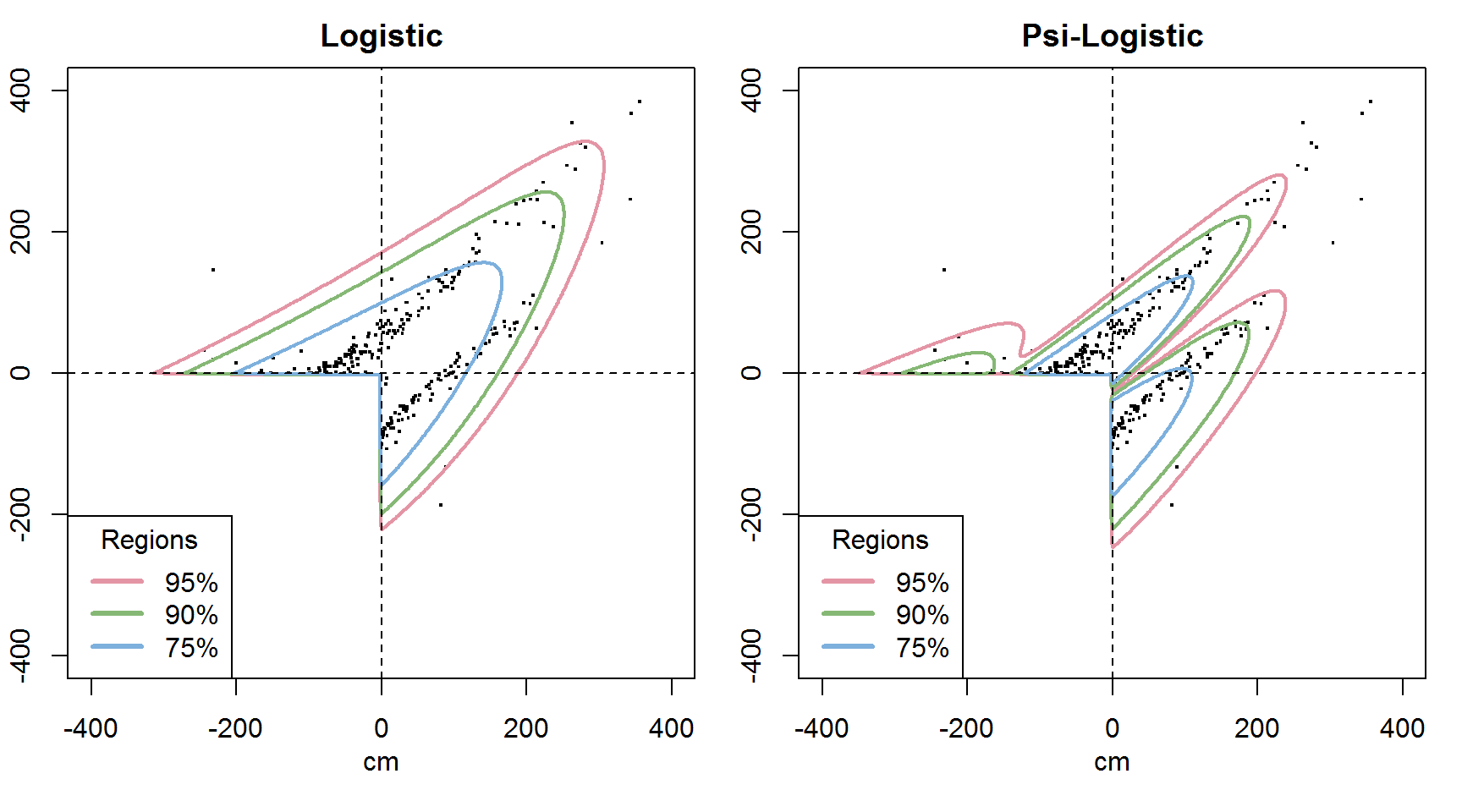# Multivariate extreme-value models

The limit distributions of coordinate-wise maxima do not form a parametric family. There are parametric models, which can be fitted by maximum likelihood. The fit should be checked by statistical tests (this question is not treated here).

There are different approaches:

• Block-maxima models (MEVD);
• Threshold models (MGPD) of different types.

The MEVD assuming unit Fréchet margins can be written as $G_{\text{Fréchet}} (t_1,\dots,t_d)= \exp \Bigl(-V (t_1,\dots,t_d) \Bigr),$ with $V (t_1,\dots,t_d)=\int_{\mathcal{S}_d} \bigvee_{i=1}^d\Big(\frac{w_i}{t_i}\Big) S(d\mathbf{w}), \label{expmeas}$ where $$S$$ is a finite measure on the $$d$$-dimensional simplex $$\mathcal{S}_d$$, satisfying the equations $\int_{\mathcal{S}_d} w_i S(d\mathbf{w})=1\; \text{ for } i=1,...,d,$ In the bivariate setting alternative formulae can be given. Let $$G$$ be a bivariate d.f. with marginals $$G_i$$. Then $-\log G(\mathbf{x}) = \ell\{-\log G_1(x_1) ,-\log G_2(x_2) \}, \qquad \mathbf{x} \in \mathbb{R}^2$ The dependence function $$A(t)=l(1-t,t), \qquad t \in [0,1]$$ satisfies the following three properties:

• $$A(t)$$ is convex,
• $$\max\{ (1-t), t\} \leq A(t) \leq 1$$
• and $$A(0) = A(1) = 1$$.

# Parametric Models

The most popular models:

• Logistic model: $\ell(v_1, v_2) = (v_1^{1/\alpha}+v_2^{1/\alpha})^\alpha,\quad v_i\geq 0,$ with parameter $$0 < \alpha \leq 1$$. Independence: $$\alpha = 1$$, complete dependence: $$\alpha \downarrow 0$$. It is symmetric, absolutely continuous. There are asymmetric generalisations.

• Negative logistic model: $\ell(v_1, v_2) = v_1+v_2-\{(\phi_1v_1)^{1/\alpha}+(\phi_2v_2)^{1/\alpha}\}^\alpha,$ where $$-\infty \leq\alpha \leq 0$$ and $$0 \leq \phi_1, \phi_2$$.

BGPD II is not absolutely continuous in the asymmetric case.

# New asymmetric models

Let $$A$$ be an arbitrary dependence function, $$\Psi(t):[0,1] \rightarrow [0,1]$$ be strictly monotonic with $$\Psi(0)=0$$ and $$\Psi(1)=1$$.

$$A_{\Psi}(t)=A(\Psi(t))$$ defines a dependence function iff

• $$A_{\Psi}(0)=A_{\Psi}(1)=0$$,
• $$(1-t)\vee t \leq A_{\Psi}(t) \leq 1$$, if $$0 \leq t \leq 1$$,
• $$A_{\Psi}'(0)=-1$$, $$A_{\Psi}'(1)=1$$,
• $$A_{\Psi}''\geq 0$$.

Let $$\Psi(t)=t+f(t)$$, e.g. $$f_{\psi_1,\psi_2}(t)=\psi_1[t(1-t)]^{\psi_2}$$, where $$\psi_1 \in \mathbb{R}$$ and $$\psi_2 \geq 2$$ are the asymmetry parameters,

Remarks

• The permitted parameter space can be determined, it is suitably rich.
• $$\psi_1=0$$ gives the original model.
• The models are absolutely continuous.
• Although the transformations $$\Psi$$ was defined now for the bivariate case only, it can be extended for the higher dimensional cases as well.

Other forms of $$f$$ are also possible, like a two-parameter family of polynomials over$$[0,1]$$ with a root in $$0<p<1$$.

# Bivariate threshold models

Bivariate (multivariate) threshold models can be defined in two different ways.

If we claim exceedance in all of the coordinates (BGPD I), we usually get simpler models, with nice properties (marginals are univariate GPD etc.), but we may use less data.

If we use all data that exceed the threshold $${\mathbf u}$$ in at least one coordinate, we get the {BGPD II} model, .

This approach can be formulated as follows. Let $$\mathbf{Y}=(Y_1,...,Y_d)$$ denote a random vector, $$\mathbf{u}=(u_1,...,u_d)$$ be a suitably high threshold vector and $$\mathbf{X} = \mathbf{Y}-\mathbf{u} = (Y_1-u_1,...,Y_d-u_d)$$ be the vector of exceedances. Then the multivariate generalized Pareto distribution (MGPD) for the $$\mathbf{X}$$ exceedances can be written by a MEVD $$G$$ with non-degenerate margins as $H({\mathbf x}) =\frac{1}{\log G\bigl(0,\dots,0 \bigr)} \log \frac{G\bigl(x_1,\dots,x_d \bigr)}{G\bigl(x_1 \wedge 0,\dots,x_d \wedge 0 \bigr)} , \label{mgpd}$ where $$0<G(0,\dots,0)<1$$. This definition provides a model for observations that are extreme in at least one component. We may switch to unit Fréchet margins by the transformation \begin{eqnarray} t_i(x_i) = \frac{-1}{\log \mathcal{G}_{\xi_i,\mu_i,\sigma_i} ( x_i )}= (1 + \xi_i (x_i - \mu_i ) / \sigma_i)^{1 / \xi_i}, \label{transf} \end{eqnarray}

with $$1 + \xi_i ( x_i - \mu_i ) / \sigma_i > 0$$ and $$\sigma_i > 0$$ for $$i=1,...,d$$.

• Remarks*

• Note if $$H_1(x)=H(x,\infty)$$ then $$H_1$$ is not a one dimensional GPD, only the conditional distribution of $$X_1|X_1>0$$ is GPD.
• All margins are dependent on all parameters, as the constant $$1/ \log G(0, . . . , 0)$$ factor remains in the formula even when $$d - 1$$ components of $$X$$ converge to infinity, so the parameters cannot be interpreted individually.
• Some models put weight to the boundaries, so they will not remain absolutely continuous.
• We can use more data, which hopefully helps in model fitting.

# Applications

We have used 100 years of daily flood peaks data from the Danube:The $$\Psi$$-logistic model follows the odd shape of the data extremely well.